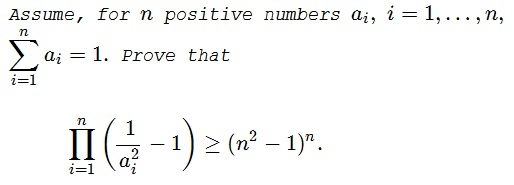# Second Pair of Twin Inequalities: Twin 1

### Problem### Solution 1

Due to the AM-GM inequality, the product $uv$ of two positive numbers with a fixed sum attains it's maximum when the numbers are equal. So the product grows as the two numbers approach each other while maintaining their sum.

It follows that, under the same convention, the expression

\displaystyle\begin{align}\left(\frac{1}{u^2}-1\right)\left(\frac{1}{v^2}-1\right)&=\frac{1-u^2-v^2+u^2v^2}{u^2v^2}\\ &=\frac{1-(u+v)^2}{(uv)^2}+\frac{2}{uv}+1 \end{align}

decreases.

Returning to the problem, if all numbers are equal to $\displaystyle \frac{1}{n},$ there is nothing to prove. Else, among positive numbers that sum up to $1,$ there bound to be one greater than $\displaystyle \frac{1}{n},$ the other less than $\displaystyle \frac{1}{n}.$ Let them move towards each other until one (or both) of them becomes $\displaystyle \frac{1}{n}.$ The product on the left of the inequality at hand may only decrease with this substitution. Continue this process while there are numbers different from $\displaystyle \frac{1}{n}.$ When there are none, i.e., when all $n$ numbers are equal, the left-hand side becomes exactly $(n^2-1)^n,$ while the original product is not less than that.

### Solution 2

Assume we have $0\lt x\le y\le 1-x.$ Then

$\displaystyle \left(\frac{1}{x}-1\right)\left(\frac{1}{y}-1\right) \ge \left(\frac{2}{x+y}-1\right)^2.$

Indeed, this is equivalent to $\displaystyle ((y - x)^2 (x + y - 1))/(x y (x + y)^2)\le 0.$ Below is an illustration with wolframalpha:Together with

$\displaystyle \left(\frac{1}{x}+1\right)\left(\frac{1}{y}+1\right) \ge \left(\frac{2}{x+y}+1\right)^2.$

we get

$\displaystyle \left(\frac{1}{x^2}-1\right)\left(\frac{1}{y^2}-1\right) \ge \left(\frac{2}{(x+y)^2}-1\right)^2.$

This justifies a step at a time process from Solution 1.

### Acknowledgment

This is problem M1272 from the Kvant magazine (1991, nn 3,8). The problem and the solution are by L. Kurlyandchik. Solution 2 is by Christopher D. Long.

### Inequalities with the Sum of Variables as a Constraint